# 開始使用輸入表單

## 編輯輸入表單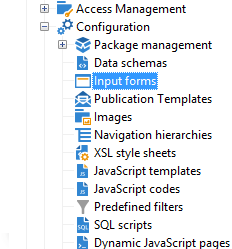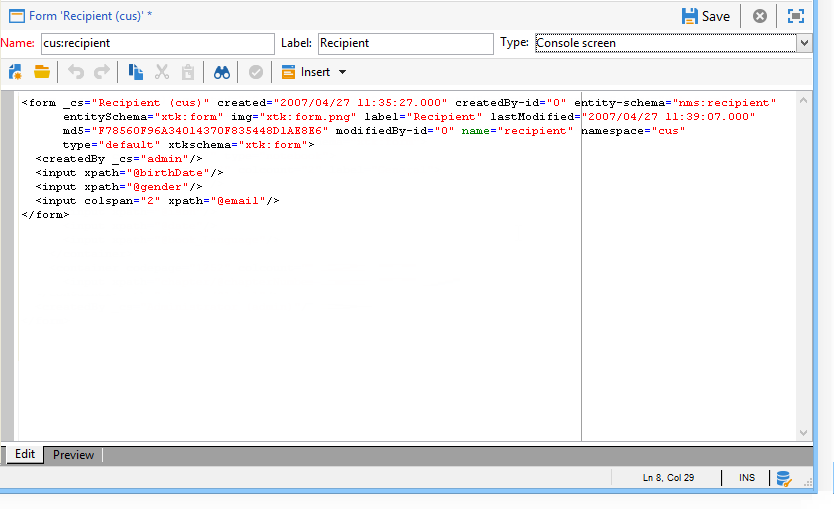## 表單結構

``````<form name="form_name" namespace="name_space">
...
</form>
``````

``````<srcSchema name="recipient" namespace="cus">
<enumeration name="gender" basetype="byte">
<value name="unknown" label="Not specified" value="0"/>
<value name="male" label="Male" value="1"/>
<value name="female" label="Female" value="2"/>
</enumeration>

<element name="recipient">
<attribute name="email" type="string" length="80" label="Email" desc="E-mail address of recipient"/>
<attribute name="birthDate" type="datetime" label="Date"/>
<attribute name="gender" type="byte" label="Gender" enum="gender"/>
</element>
</srcSchema>
````````````<form name="recipient" namespace="cus">
<input xpath="@gender"/>
<input xpath="@birthDate"/>
<input xpath="@email"/>
</form>
``````

`<input label="E-mail address" xpath="@name" />`所有表單屬性都列在 Campaign Classicv7文檔

## 格式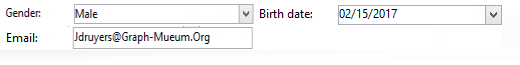``````<form name="recipient" namespace="cus">
<container colcount="2">
<input xpath="@gender"/>
<input xpath="@birthDate"/>
<input xpath="@email"/>
</container>
</form>
``````

`<container>` 元素 計數 屬性用於將子控制項的顯示強制到兩列。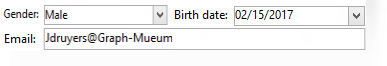``````<form name="recipient" namespace="cus">
<container colcount="2">
<input xpath="@gender"/>
<input xpath="@birthDate"/>
<input xpath="@email" colspan="2"/>
</container>
</form>
``````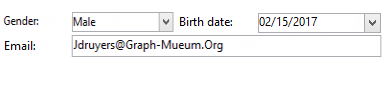``````<form name="recipient" namespace="cus">
<container colcount="2" type="frame" label="General">
<input xpath="@gender"/>
<input xpath="@birthDate"/>
<input xpath="@email" colspan="2"/>
</container>
</form>
``````

A `<static>` 元素可用於格式化輸入表單：``````<form name="recipient" namespace="cus">
<static type="separator" colspan="2" label="General"/>
<input xpath="@gender"/>
<input xpath="@birthDate"/>
<input xpath="@email" colspan="2"/>
<static type="help" label="General information about recipient with date of birth, gender, and e-mail address." colspan="2"/>
</form>
``````

`<static>` 標籤 分離器 「類型」(Type)，用於添加包含標籤的分隔條 標籤 屬性。

## 使用容器

xpath 屬性 `<container>` 用於簡化子控制項的引用。 然後，控制項的引用相對於父項 `<container>` 父項。

``````<container colcount="2">
<input xpath="location/@zipCode"/>
<input xpath="location/@city"/>
</container>
``````

``````<container colcount="2" xpath="location">
<input xpath="@zipCode"/>
<input xpath="@city"/>
</container>
``````

### 添加頁籤（筆記本）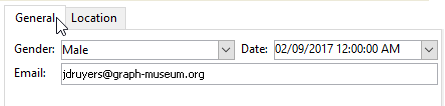``````<container type="notebook">
<container colcount="2" label="General">
<input xpath="@gender"/>
<input xpath="@birthDate"/>
<input xpath="@email" colspan="2"/>
</container>
<container colcount="2" label="Location">
...
</container>
</container>
```````<container style="down" type="notebook"> ... </container>`

### 添加表徵圖（表徵圖框）``````<container type="iconbox">
<container colcount="2" label="General" img="xtk:properties.png">
<input xpath="@gender"/>
<input xpath="@birthDate"/>
<input xpath="@email" colspan="2"/>
</container>
<container colcount="2" label="Location" img="nms:msgfolder.png">
...
</container>
</container>
``````

### 隱藏容器(visibleGroup)

``````<container type="visibleGroup" visibleIf="@gender=1">
...
</container>
<container type="visibleGroup" visibleIf="@gender=2">
...
</container>
``````

• visibleIf="@email='peter.martinezATneeolane.net'":test字串類型資料的等式。 比較值必須用引號表示。
• visibleIf="@gender >= 1和@gender != 2":的子菜單。
• visibleIf="@boolean1=true或@boolean2=false":test布爾欄位。

### 條件顯示(enabledGroup)

``````<container type="enabledGroup" enabledIf="@gender=1">
...
</container>
<container type="enabledGroup" enabledIf="@gender=2">
...
</container>
``````

``````<element label="Company" name="company" target="cus:company" type="link"/>
``````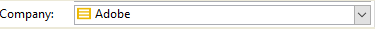``````<input xpath="company"/>
``````

Edit link （放大鏡）表徵圖將啟動連結元素的編輯窗體。 預設情況下，在目標模式的鍵上推導所用格式。 的 表格 屬性用於強制編輯表單的名稱(例如"cus:company2")。

``````<input xpath="company">
<sysFilter>
<condition expr="[location/@city] =  'Newton"/>
</sysFilter>
</input>
``````

``````<input xpath="company">
<orderBy>
<node expr="[location/@zipCode]"/>
</orderBy>
</input>
``````

## 控制項屬性

• 無自動完成:禁用超前類型（使用值"true"）

• 建立模式:如果連結不存在，則即時建立該連結。 可能的值為：

• :禁用建立。 如果連結不存在，則顯示錯誤消息
• :建立與編輯欄位中的內容的連結
• 版本:顯示連結上的編輯窗體。 驗證表單後，將保存資料（預設模式）
• noZoom:連結上沒有編輯窗體（值為"true"）

• 表格:重載目標元素的編輯窗體

``````<element label="Events" name="rcpEvent" target="cus:event" type="link" unbound="true">
...
</element>
``````

`````` <input xpath="rcpEvent" type="linklist">
<input xpath="@label"/>
<input xpath="@date"/>
</input>
``````

Detail 按鈕 zoom="true" 屬性在上完成 `<input>` 清單的標籤：它允許您啟動選定行的編輯窗體。

`````` <input xpath="rcpEvent" type="linklist">
<input xpath="@label"/>
<input xpath="@date"/>
<sysFilter>
<condition expr="@type = 1"/>
</sysFilter>
<orderBy>
<node expr="@date" sortDesc="true"/>
</orderBy>
</input>
``````

## 定義關係表

``````<srcSchema name="subscription" namespace="cus">
<element name="service" type="link" target="cus:service" label="Subscription service"/>
</srcSchema>
``````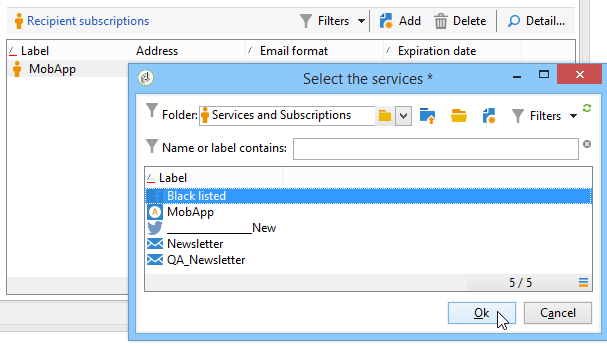``````<input type="linklist" xpath="subscription" xpathChoiceTarget="service" xpathEditTarget="service" zoom="true">
<input xpath="recipient"/>
<input xpath="service"/>
</input>
``````

xpathChoiceTarget 屬性允許您從輸入的連結啟動選擇表單。 建立關係表記錄將自動更新指向當前收件人和所選服務的連結。

xpathEditTarget 屬性用於在輸入的連結上強制編輯選定行。

### 清單屬性

• 無工具欄:隱藏工具欄（值為"true"）
• 工具欄標題:重載工具欄標籤
• 工具欄對齊:修改工具欄的垂直或水準幾何(可能的值："垂直"|"水準"
• img:顯示與清單關聯的影像
• 表格:重載目標元素的編輯窗體
• 縮放:添加 Zoom 按鈕以編輯目標元素
• xpathEditTarget:設定輸入的連結的編輯
• xpathChoiceTarget:另外，在輸入的連結上啟動選擇表單

## 添加列清單

``````<input xpath="rcpEvent" type="list">
<input xpath="@label"/>
<input xpath="@date"/>
</input>
``````

``````<input nolabel="true" toolbarCaption="List of events" type="list" xpath="rcpEvent" zoom="true">
<input xpath="@label"/>
<input xpath="@date"/>
</input>
``````

### 啟用放大清單

``````<input nolabel="true" toolbarCaption="List of events" type="list" xpath="rcpEvent" zoom="true" zoomOnAdd="true">
<input xpath="@label"/>
<input xpath="@date"/>

<form colcount="2" label="Event">
<input xpath="@label"/>
<input xpath="@date"/>
</form>
</input>
``````

### 清單屬性

• 無工具欄:隱藏工具欄（值為"true"）
• 工具欄標題:重載工具欄標籤
• 工具欄對齊:修改工具欄的位置(可能的值："垂直"|"水準"
• img:顯示與清單關聯的影像
• 表格:重載目標元素的編輯窗體
• 縮放:添加 Zoom 按鈕以編輯目標元素
• xpathChoiceTarget:另外，在輸入的連結上啟動選擇表單

## 添加不可編輯的欄位

"性別"欄位示例：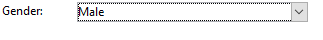``````<value value="@gender"/>
``````

## 添加單選按鈕

"性別"欄位示例：

``````<input type="RadioButton" xpath="@gender" checkedValue="0" label="Choice 1"/>
<input type="RadioButton" xpath="@gender" checkedValue="1" label="Choice 2"/>
<input type="RadioButton" xpath="@gender" checkedValue="2" label="Choice 3"/>
``````## 添加複選框

``````<input xpath="@boolean1"/>
<input xpath="@field1" type="checkbox" checkedValue="Y"/>
``````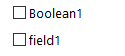``````<input nolabel="true" type="treeEdit">
<container label="Text fields">
<input xpath="@text1"/>
<input xpath="@text2"/>
</container>
<container label="Boolean fields">
<input xpath="@boolean1"/>
<input xpath="@boolean2"/>
</container>
</input>
``````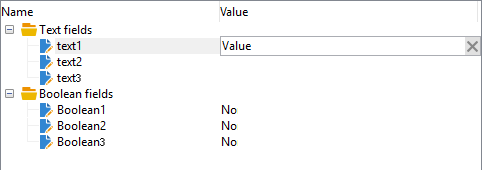## 添加表達式欄位

``````<!-- Example: updating the boolean1 field from the value contained in the field with path /tmp/@flag -->
<input expr="Iif([/tmp/@flag]=='On', true, false)" type="expr" xpath="@boolean1"/>
<input expr="[/ignored/@action] == 'FCP'" type="expr" xpath="@launchFCP"/>
``````

## 表單上下文

### 更新上下文

• `<set expr="'Test'" xpath="/tmp/@test" />`:將「Test」值定位在臨時位置/tmp/@test1
• `<set expr="'Test'" xpath="@lastName" />`:使用「Test」值更新「lastName」屬性上的實體
• `<set expr="true" xpath="@boolean1" />`:將"boolean1"欄位的值設定為"true"
• `<set expr="@lastName" xpath="/tmp/@test" />`:更新"lastName"屬性的內容

``````<form name="recipient" namespace="cus">
<enter>
<set...
</enter>
...
<leave>
<set...
</leave>
</form>
``````

`<enter>``<leave>` 標籤可用於 `<container>` 頁（「筆記本」和「表徵圖框」類型）。

### 表達語言

`<if expr="<expression>" />` 如果驗證了表達式，則標籤將執行標籤下指定的指令：

``````<if expr="([/tmp/@test] == 'Test' or @lastName != 'Doe') and @boolean2 == true">
<set xpath="@boolean1" expr="true"/>
</if>
``````

`<check expr="<condition>" />``<error>` 標籤會阻止對表單的驗證，並在條件不滿足時顯示錯誤消息：

``````<leave>
<check expr="/tmp/@test != ''">
<error>You must populate the 'Test' field!</error>
</check>
</leave>
``````

## 助理（嚮導）

``````<form type="wizard" name="example" namespace="cus" img="nms:rcpgroup32.png" label="Wizard example" entity-schema="nms:recipient">
<container title="Title of page 1" desc="Long description of page 1">
<input xpath="@lastName"/>
<input xpath="comment"/>
</container>
<container title="Title of page 2" desc="Long description of page 2">
...
</container>
...
</form>
``````

Finish 按鈕保存輸入的資料並關閉窗體。

### SOAP方法

`<soapcall>` tag包含對具有以下輸入參數的方法的調用：

``````<soapCall name="<name>" service="<schema>">
<param type="<type>" exprIn="<xpath>"/>
...
</soapCall>
``````

`<param>` 元素 `<soapcall>` 標籤。

• 字串:字串
• 布爾:布爾型
• 位元組:8位整數
• :16位整數
• :32位整數
• :16位整數
• :雙精度浮點數
• DOMElement:元素型節點

exprIn 屬性包含要作為參數傳遞的資料的位置。

``````<leave>
<soapCall name="RegisterGroup" service="nms:recipient">
<param type="DOMElement" exprIn="/tmp/entityList"/>
<param type="DOMElement" exprIn="/tmp/choiceList"/>
<param type="boolean"    exprIn="true"/>
</soapCall>
</leave>
``````